# 数据库导论 关系数据库理论

## DROP 'theory' FROM 'dbKnowledgeBase';

Posted by R1NG on January 11, 2022 Viewed Times

# COMP23111 REVISION Ch1

## 1. 数据库理论

CREATE, ALTER, DROP, RENAME 等建表相关的語句為 数据定义语言 DDL, 也就是 Data Definition Language; INSERT, UPDATE, DELETE 等直接对 数据 进行操作的语句为 数据操作语言 (DML, Database Manipulation Language), 以 SELECT 语句为例的, 用于执行信息查询的语句为 数据库查询语言 (DQL, Data Query Language). SQL 是由上述三种语句组合而成的.

### 1.2 实体-关系 (E-R) 模型

(Key) 定义为 在实体-关系模型中可用于唯一确定 (Uniquely Identify) 某个实体的属性, 也就是说每个不同实体的这个属性值都不相同.

E-R 模型通常使用的属性类型有: 简单/复合属性, 单值/多值属性, 存储/派生属性:

### 1.3 数据库的关系模型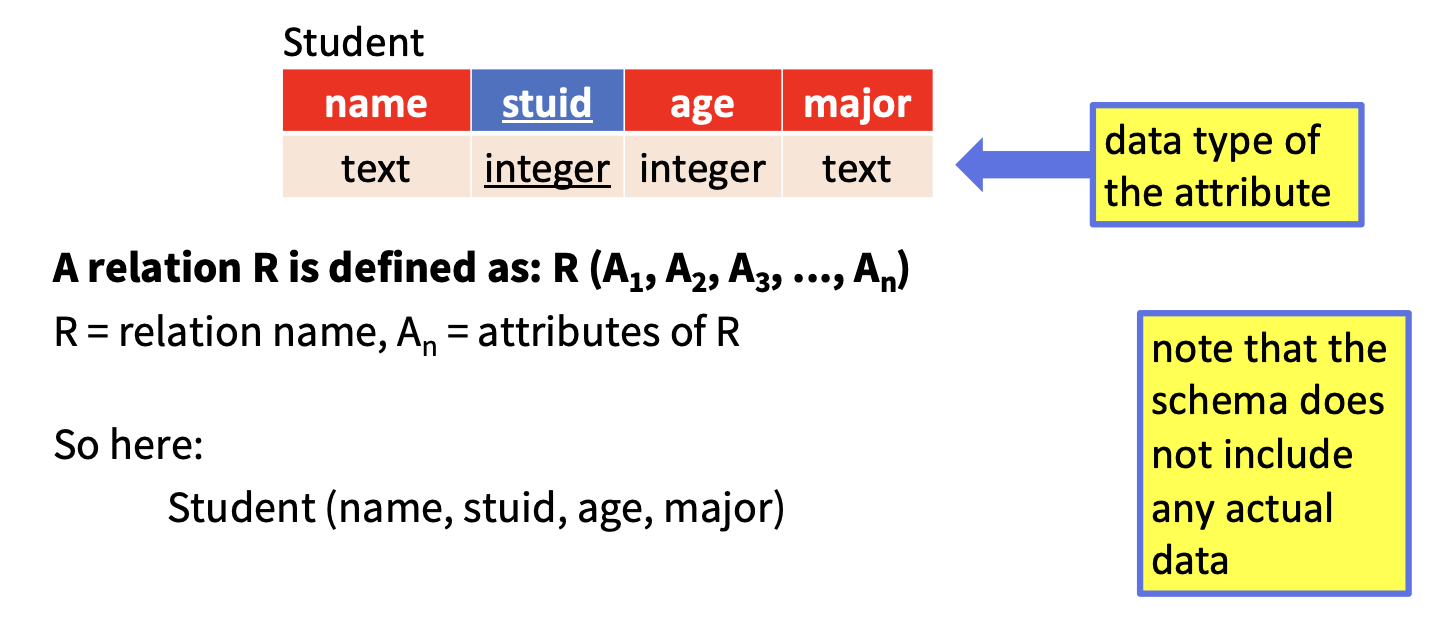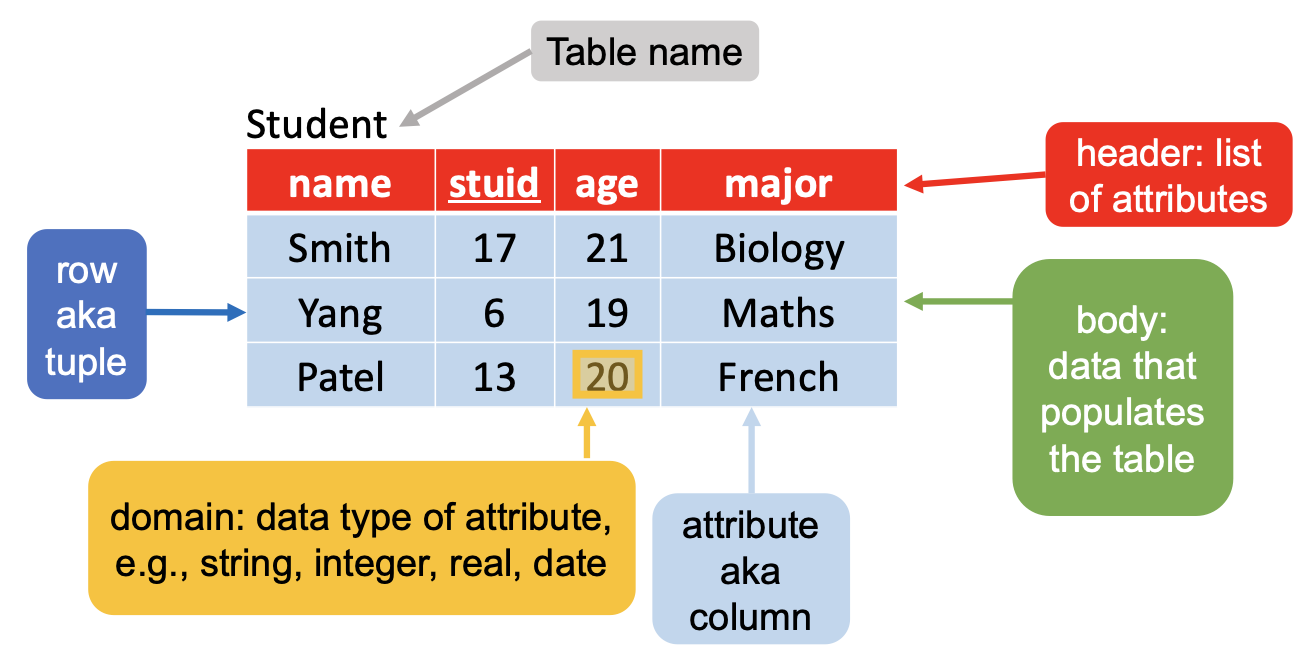$R(A_1, A_2, \cdots, A_n),$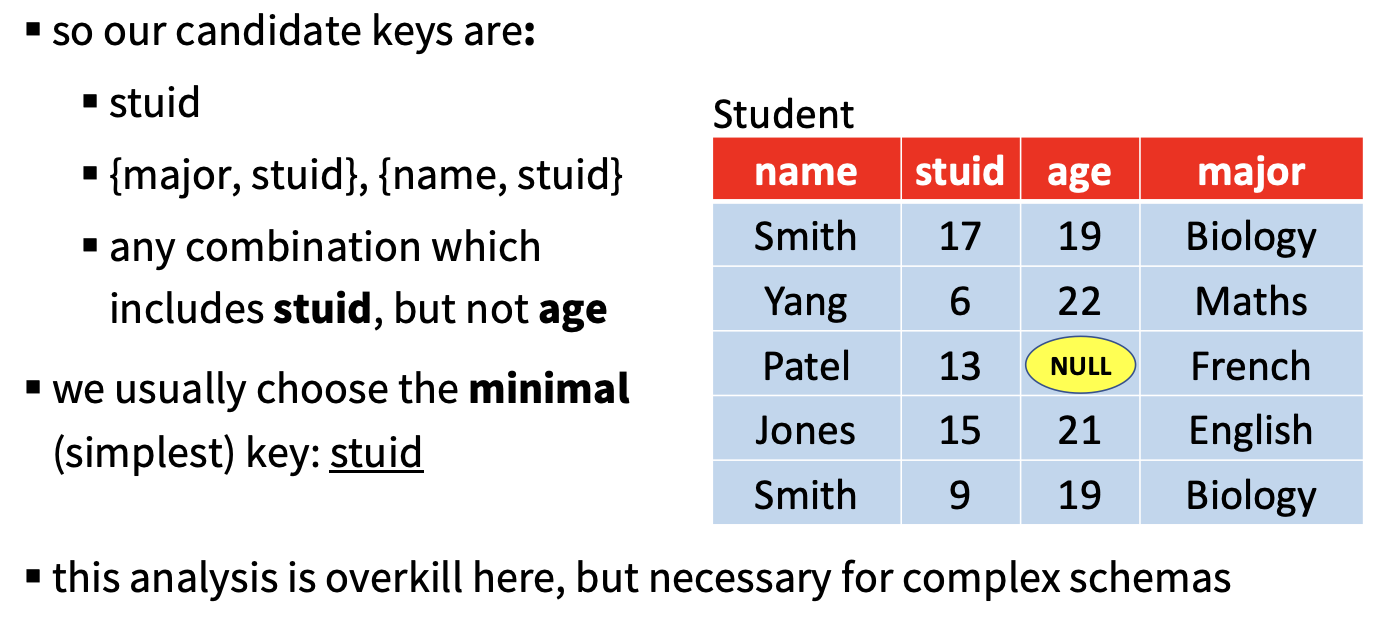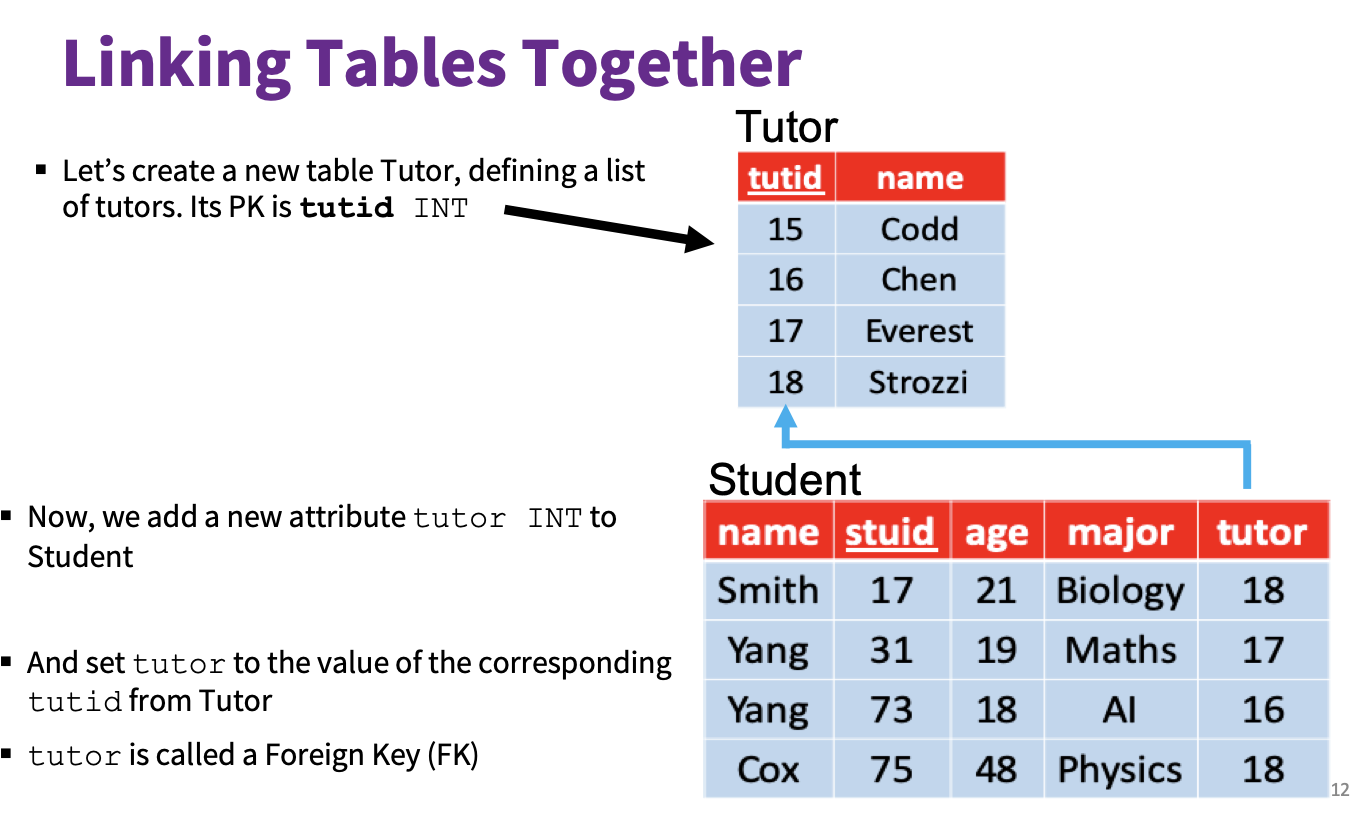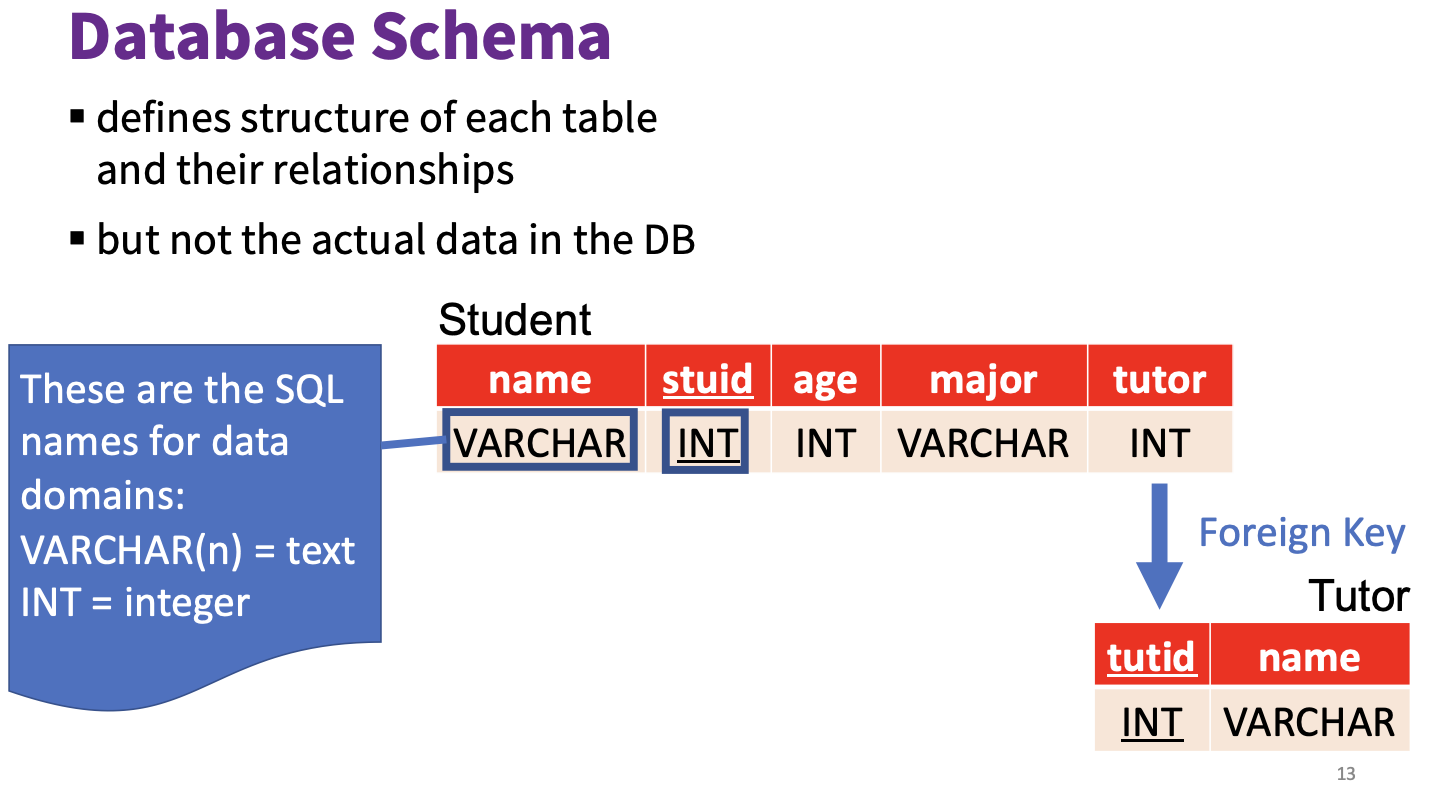1. 主键约束 (Primay Key Constraint): 确保数据字段的 唯一性非空性.

2. 唯一约束 (Unique Constraint): 确保数据字段的 唯一性. (但是可为空)

3. 检查约束 (Check Constraint): 限制该数据字段的范围和格式.

4. 默认约束 (Default Constraint) 赋予该数据字段规定好的默认值.

5. 外键约束 (Foreign Key Constraint): 需要建立两表间的关系并引用主表的列.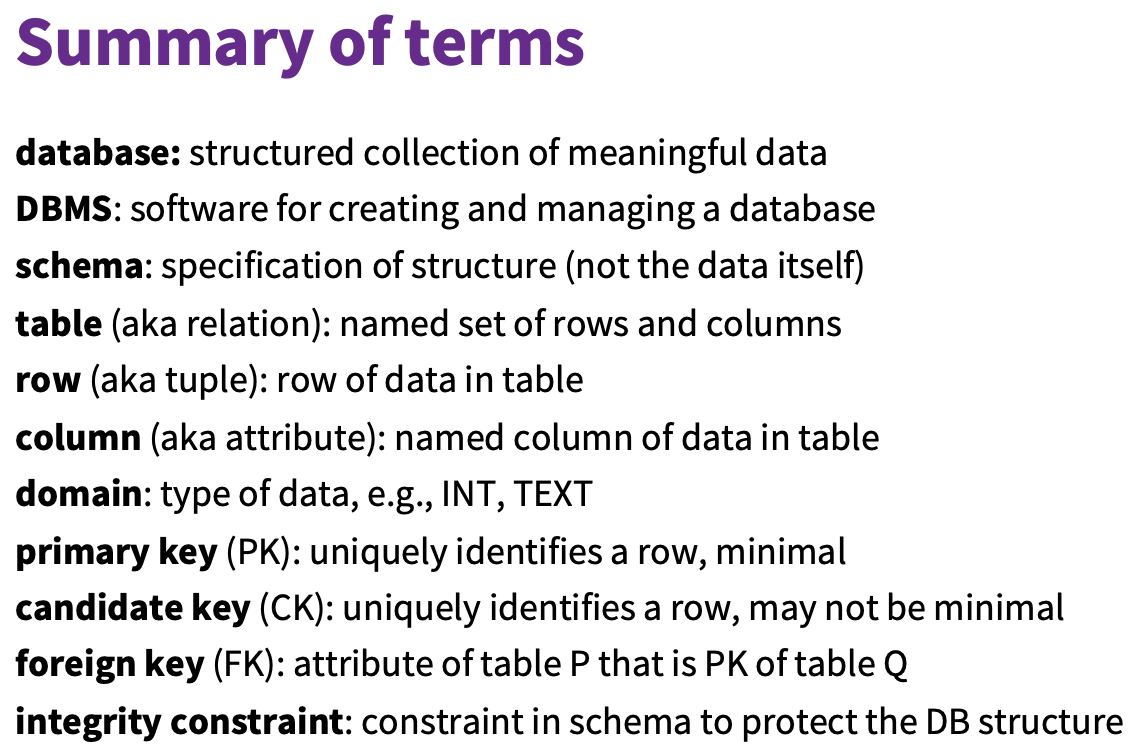### 1.4 函数依赖 (Functional Dependencies) 和范式 (Normal Form)

$X \rightarrow Y.$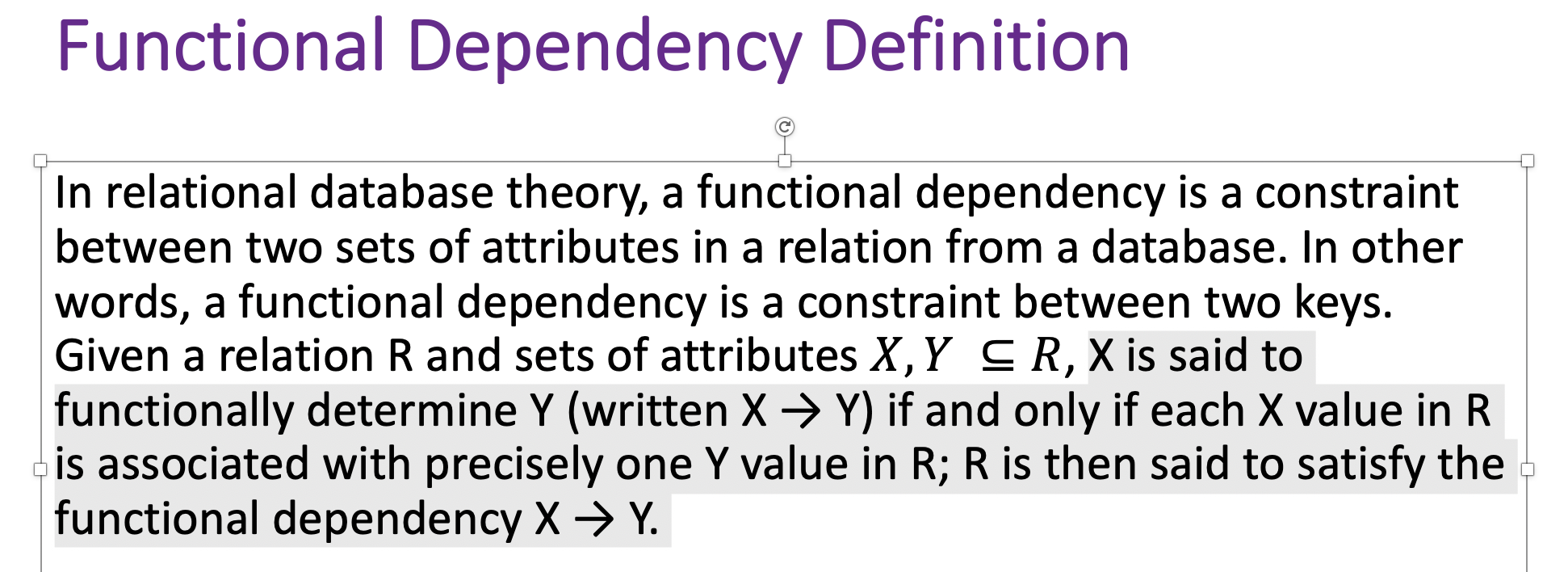$X' \nrightarrow Y$

$X \overset{F}{\rightarrow} Y.$

$X \overset{P}{\rightarrow} Y.$

1. $Y$ 函数依赖于 $X$;
2. $Z$ 函数依赖于 $Y$,

$X \overset{T}{\rightarrow} Z.$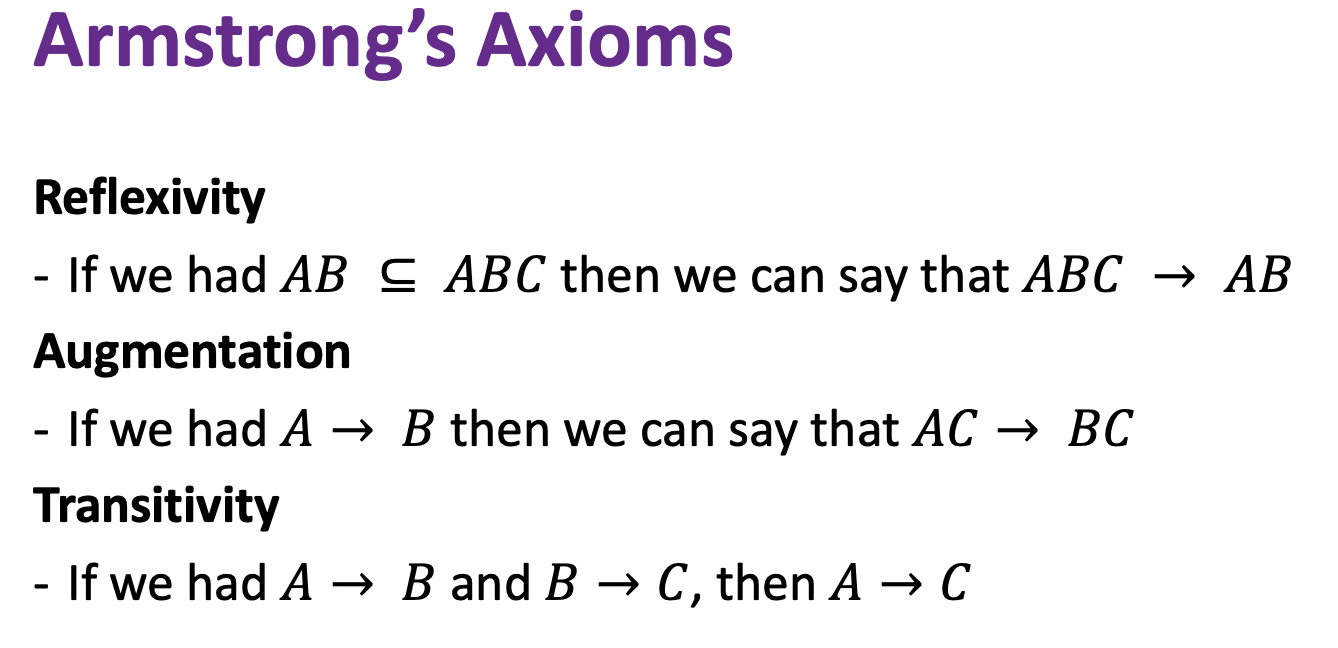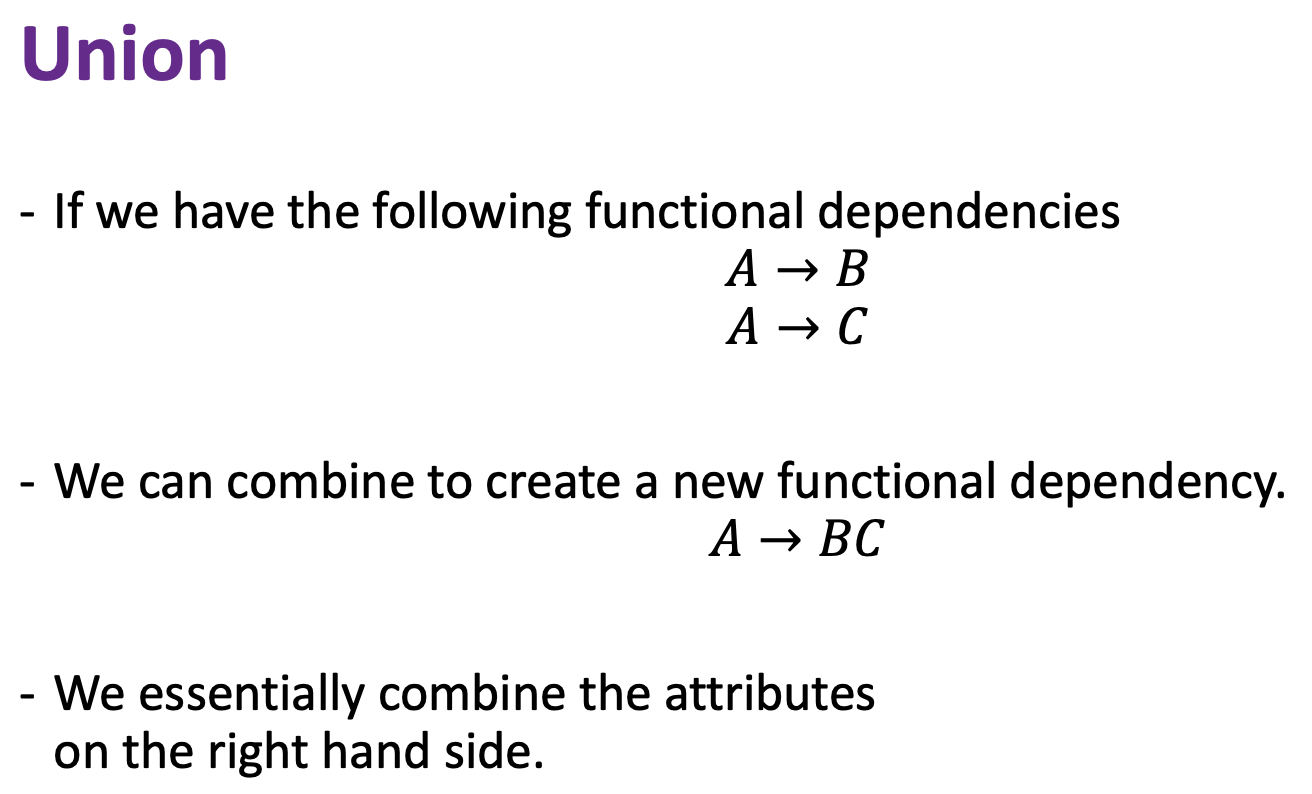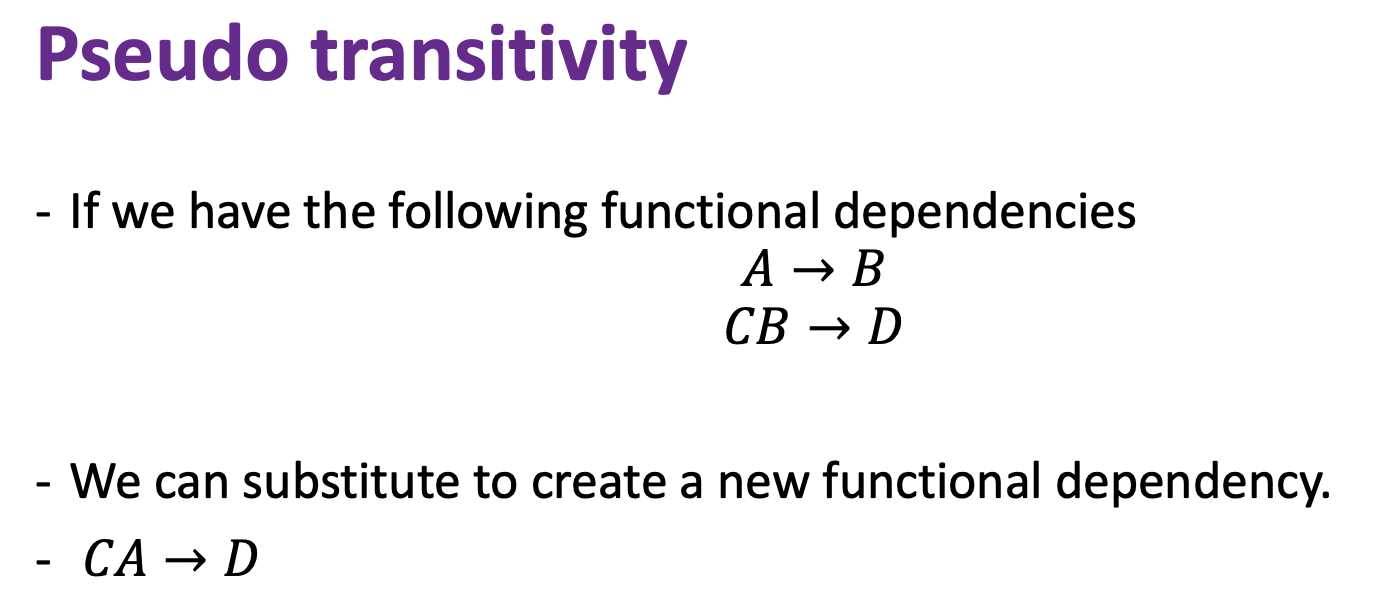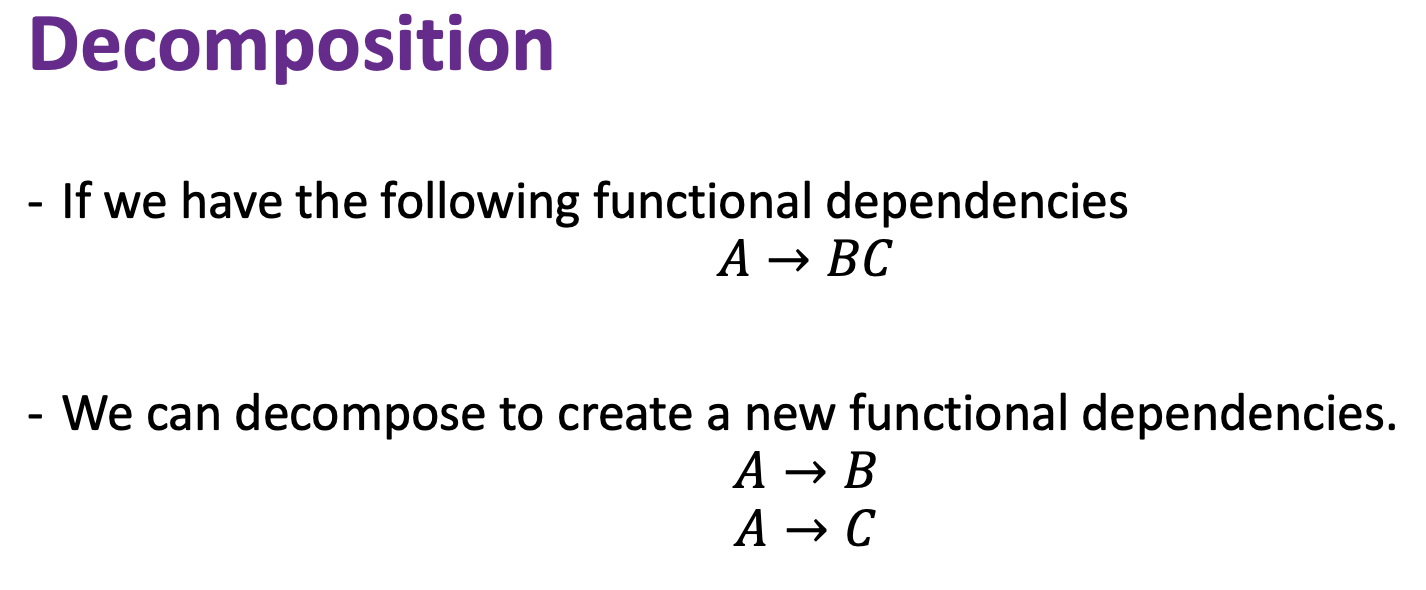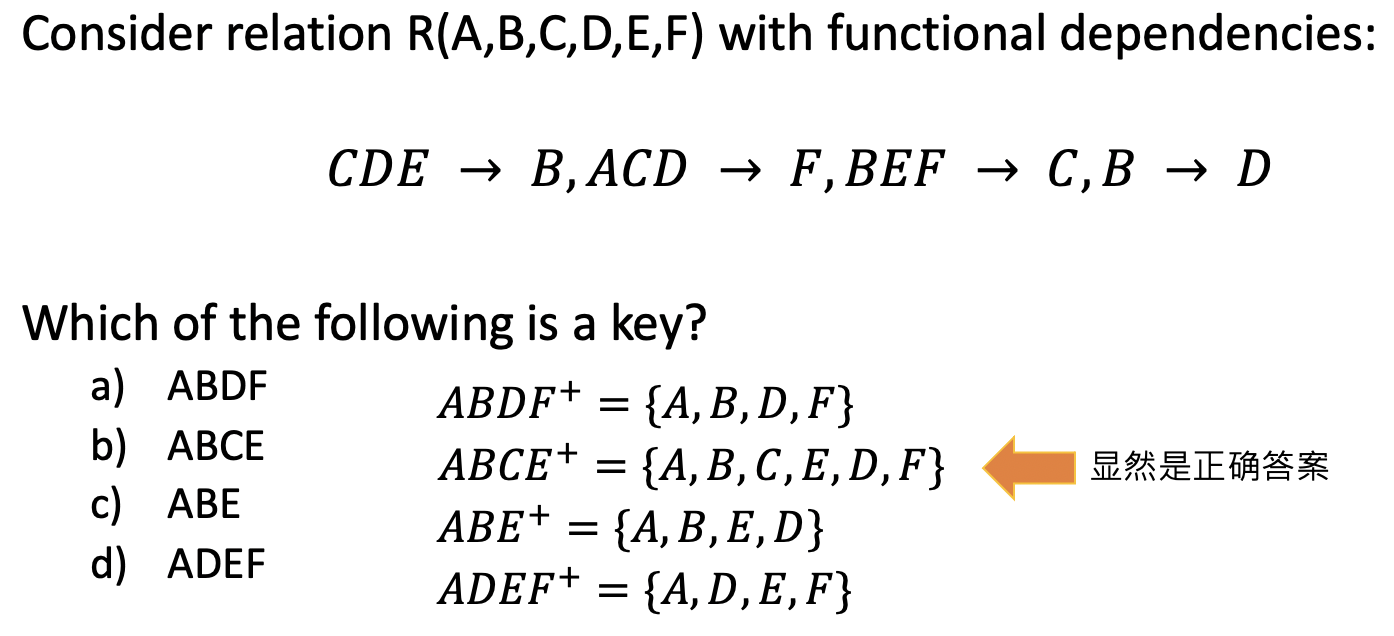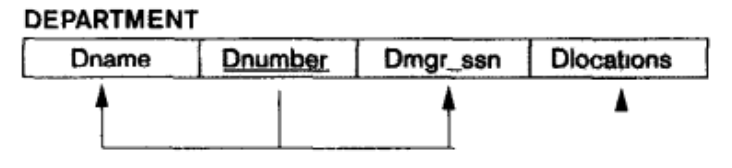1. 找到那些部分依赖主键的属性.

2. 确定它们分别 完全依赖于主键的哪个组成部分.

3. 将原来的关系进行拆分, 每个部分依赖主键的属性都要被从原关系中拆分出来, 和它所完全依赖的那一部分主键组合在一起形成一个满足 2NF 的, 更小的关系.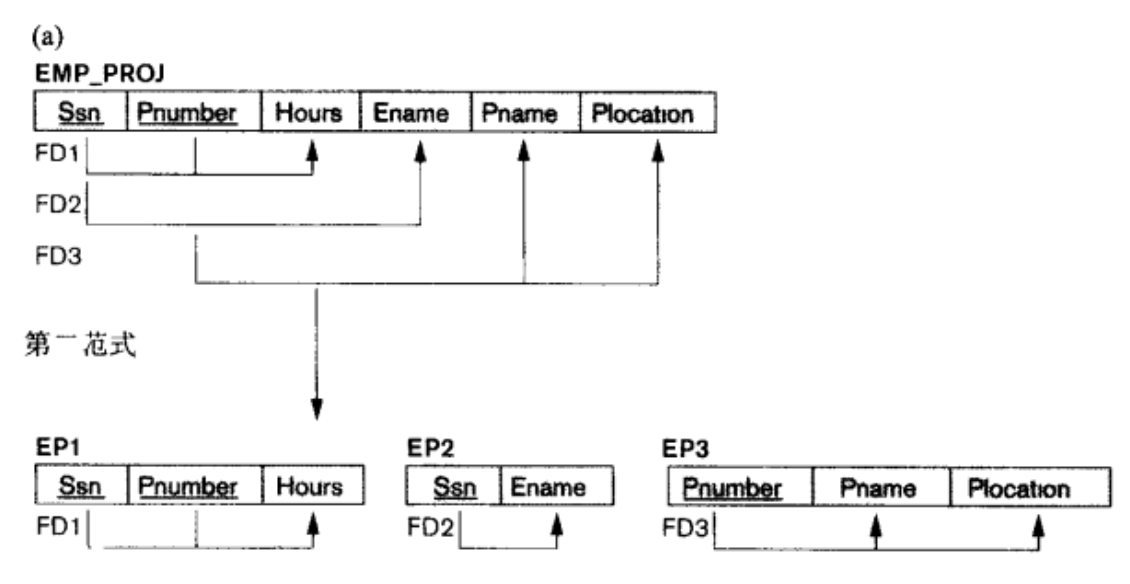1. $X \rightarrow Y$
2. $Y \rightarrow Z$

1. 找到间接依赖主键的属性链条.

2. 拆分关系, 打散链条.对于间接依赖链条上除了主键以外的每个节点, 都要单独分解为 3NF 模式进行规范化.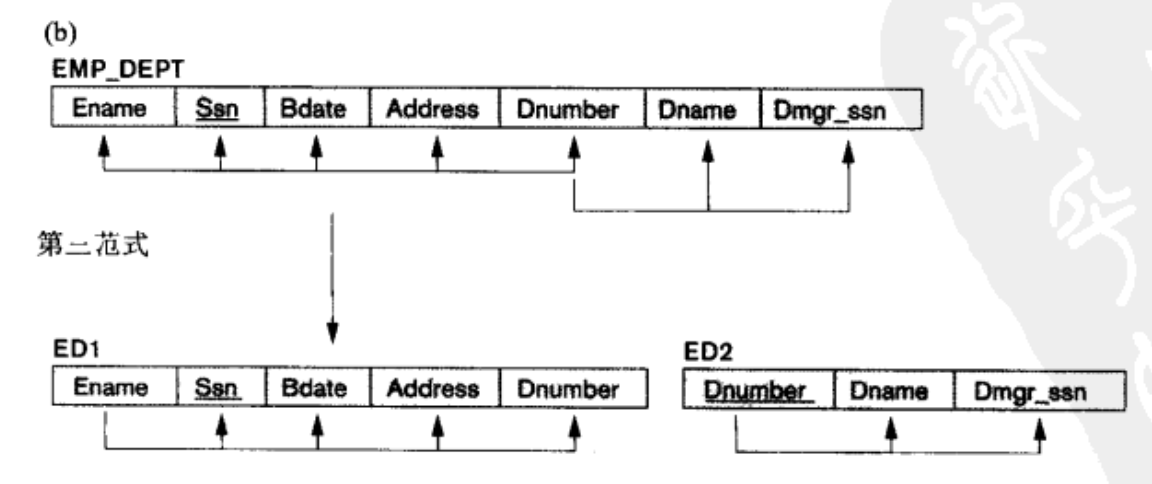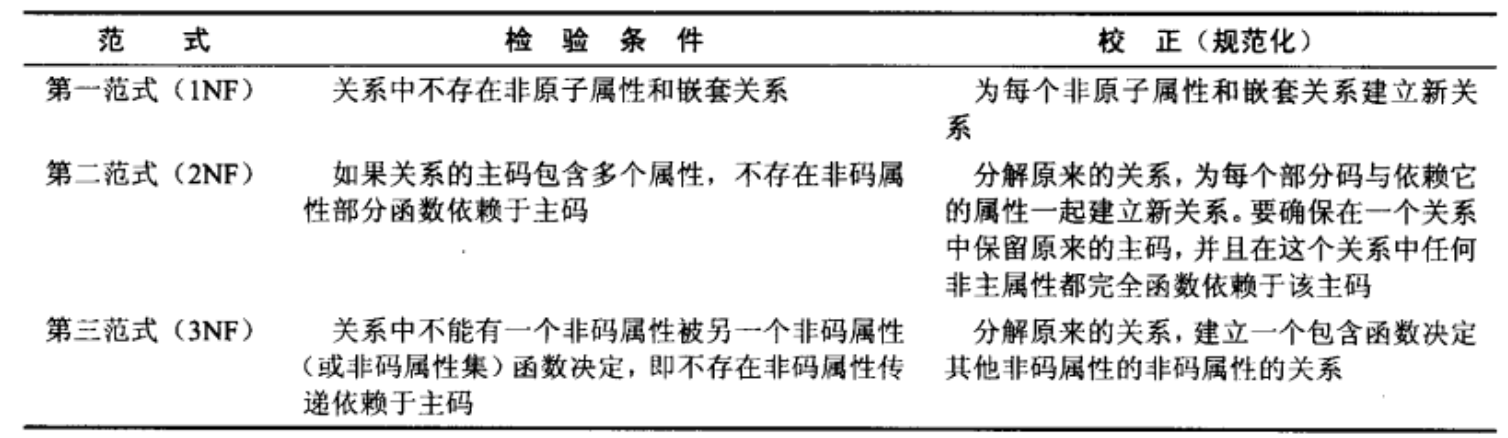REFERENCE

Entity Relationship Diagram (ERD) Tutorial - Part 1

Entity Relationship Diagram (ERD) Tutorial - Part 2

Wikipedia: Conceptual schema